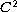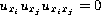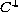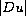Electron. J. Diff. Eqns., Vol. 2006(2006), No. 122, pp. 1-4.

### A remark oninfinity-harmonic functions Yifeng Yu

Abstract:
In this paper, we prove that any nonconstant,solution of the infinity Laplacian equationcan not have interior critical points. This result was first proved by Aronsson  in two dimensions. When the solution is, Evans  established a Harnack inequality for, which implies that non-constantsolutions have no interior critical points for any dimension. Our method is strongly motivated by the work in .

Submitted June 15, 2006. Published October 6, 2006.
Math Subject Classifications: 35B38.
Key Words: Infinity Laplacian equation; infinity harmonic function; viscosity solutions.

Show me the PDF file (170K), TEX file, and other files for this article.Yifeng Yu Department of Mathematics University of Texas Austin, TX 78712, USA email: yifengyu@math.utexas.edu